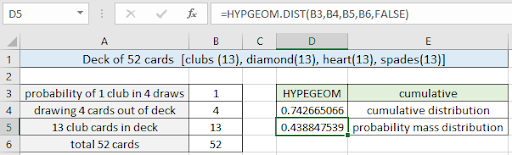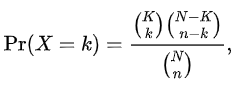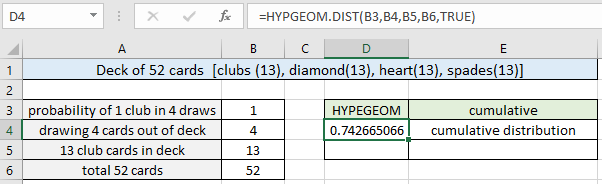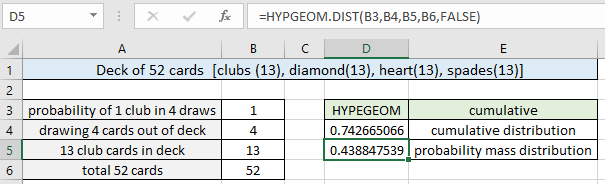# How to use the HYPGEOM.DIST function in ExcelIn this article, we will learn How to use the HYPGEOM.DIST function in Excel.

What is hypergeometric distribution ?

Hypergeometric distribution is a discrete probability distribution that describes the probability of k successes in a sample without replacement from a finite population. For example drawing 4 balls from a bag of 20 balls containing 8 white and 12 black balls. What is the probability of drawing exactly 1 white ball out of the 4 balls drawn without replacement. You must be thinking how this question is different from the normal distribution function. The problem occurs for the condition of without replacement. When we replace the drawn ball, the probability of the drawn ball remains same for each event whereas if we don't replace the ball, the probability of ball changes in the bag for each draw. Mathematically, we have a formula to calculate probability for such cases.Here :

N is the population size,

K is the number of success states in the population,

n is the number of draws (i.e. quantity drawn in each trial),

k is the number of observed successes,

(  ) is a combinator function expressed as n! / (k! * (n-k)!) (here ! is the factorial function)

This is still very complex to use in Excel. For this Excel provides the built in HYPGEOM.DIST function to calculate probability value in geometric distribution without replacement.

HYPGEOM.DIST Function in Excel

HYPGEOM.DIST takes the 4 numerical argument and 1 boolean value which determines the type of probability function (cdf or pdf). Let's understand these arguments one by one listed below.

HYPGEOM.DIST Function syntax:

 =HYPGEOM.DIST ( sample_s , number_sample , population_s , number_pop , cumulative)

sample_s : number of successes in the sample
number_sample : sample size drawn

population_s : number of success in the population

number_pop : population size

cumulative : logical value that determines the form of the function. If cumulative is TRUE, HYPGEOM.DIST returns the cumulative distribution function; if FALSE, it returns the probability density function.

Example :

All of these might be confusing to understand. Let's understand how to use the function using an example. Here we take a regular deck of cards and we draw 4 cards from the deck without replacement. What is the probability of getting exactly 1 club card in the drawn 4 cards? Let's use the below formula to get the required probability.

Use the formula:

 =HYPGEOM.DIST( B3, B4, B5, B6, TRUE)As you can see, there is a 0.74 or 74% (approx) probability of getting exactly 1 club card when drawing 4 cards without replacement.

Now we can calculate the probability for drawing at least 1 club card for the same parameters just by changing the cumulative argument in the function shown below.

Use the formula:

 =HYPGEOM.DIST( B3, B4, B5, B6, FALSE)As you can see, there is a 0.44 or 44% (approx) probability of getting at least 1 club card when drawing 4 cards without replacement. You can use the BINOM.DIST function, if drawn cards are replaced back as BINOM.DIST function works on the condition that probabilities must remain for the drawn cards.

Here are all the observational notes using the HYPGEOM.DIST function in Excel
Notes :

1. The function only works with numbers. If any argument other than cumulative is non numeric, the function returns #VALUE! error.
2. The function returns #NUM! Error.
1. If sample_s value is < 0 or sample_s value > number_sample.
2. If sample_s value > population_s value.
3. If sample_s value > (number_sample - number_pop + population_s) value.
4. If Number_sample value ? 0 or Number_sample > number_pop.
5. If population_s value ? 0 or population_s > number_pop.
6. If number_pop ? 0
3. The cumulative argument can be used with boolean numbers (0 and 1) or (FALSE or TRUE).
4. Value in decimal or value in percentage is the same value in Excel. Convert the value to percentage, if required.
5. You can feed the arguments to the function directly or using the cell reference as explained in the example.

Hope this article about How to use the HYPGEOM.DIST function in Excel is explanatory. Find more articles on statistical formulas and related Excel functions here. If you liked our blogs, share it with your friends on Facebook. And also you can follow us on Twitter and Facebook. We would love to hear from you, do let us know how we can improve, complement or innovate our work and make it better for you. Write to us at info@exceltip.com.

Related Articles :

How To Use Excel T TEST Function in Excel : The T.TEST function is used to determine the confidence of an analysis in Excel. Mathematically, it is used to know if the mean of the two samples are equal or not. T.TEST is used to accept or reject the null hypothesis.

How to use Excel F.TEST Function in Excel : The F.TEST Function is used to calculate F statistic of two samples in excel internally and returns the two tailed probability of the F statistic under Null Hypothesis.

How to use the DEVSQ Function in Excel : DEVSQ function is a built-in statistical function to calculate the sum of squared deviations from the mean or average of the given distribution provided.

How to use Excel NORM.DIST Function : Calculate the Z score for the normal cumulative distribution for the pre specified values using the NORMDIST function in Excel.

How to use Excel NORM.INV Function : Calculate the inverse of Z score for the normal cumulative distribution for the pre-specified probability values using the NORM.INV function in Excel.

How to calculate Standard Deviation in Excel : To calculate the standard deviation we have different functions in Excel. The standard deviation is the square root of the variance value but It tells more about the dataset than variance.

How to use the VAR function in Excel : Calculate the variance for the sample dataset in excel using the VAR function in Excel.

Popular Articles :

How to use the IF Function in Excel : The IF statement in Excel checks the condition and returns a specific value if the condition is TRUE or returns another specific value if FALSE.

How to use the VLOOKUP Function in Excel : This is one of the most used and popular functions of excel that is used to lookup value from different ranges and sheets.

How to use the SUMIF Function in Excel : This is another dashboard essential function. This helps you sum up values on specific conditions.

How to use the COUNTIF Function in Excel : Count values with conditions using this amazing function. You don't need to filter your data to count specific values. Countif function is essential to prepare your dashboard.

Terms and Conditions of use

The applications/code on this site are distributed as is and without warranties or liability. In no event shall the owner of the copyrights, or the authors of the applications/code be liable for any loss of profit, any problems or any damage resulting from the use or evaluation of the applications/code.